# Equations of Mathematical Physics

The following article is from The Great Soviet Encyclopedia (1979). It might be outdated or ideologically biased.

## Equations of Mathematical Physics

the partial differential equations and some related equations of other types (such as integral and integro-differential equations) to which the mathematical analysis of physical phenomena leads.

The formulation of problems in the form required in the investigation of physical phenomena is characteristic of the theory of equations of mathematical physics. The range of equations of mathematical physics has been undergoing a steady expansion with the application of mathematical analysis to new areas. The systematization of obtained results requires that the theory of equations of mathematical physics include equations and problems of a more general form than those that appear in the analysis of specific phenomena. It is characteristic, however, even of such general equations and problems that their properties allow a more or less obvious physical interpretation (see).

Classification. Many equations of mathematical physics are second-order linear partial differential equations of the general form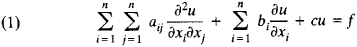Here, the coefficients aij (aij = aij), bi, c and the right-hand side f are given functions of the independent variables x1, x2, . . ., xn (n ≥ 2), and u is an unknown function of the same independent variables.

The properties of the solutions of equation (1) depend essentially on the signs of the roots of the equation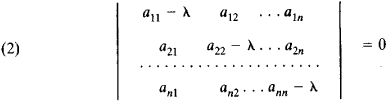which is algebraic with respect to λ. Equations (1) are therefore classified into types in accordance with these signs. If all n roots of equation (2) have the same sign, then equation (1) is said to be elliptic. If one of the roots is opposite in sign to the remaining n – 1 roots, then equation (1) is hyperbolic. Finally, if one root is zero and the other roots are of the same sign, then equation (1) is parabolic.

If the coefficients aij are constant, then equation (1) is of a specific type regardless of the values of the independent variables. On the other hand, if these coefficients depend on x1, ..., xn, then the roots of equation (2) also depend on x1, . . ., xn, and equation (1) may therefore be of different types for different values of the independent variables. In this case the equation is said to be of mixed type, and the investigated range of the independent variables consists of regions in which the type of equation (1) does not change. If the root of equation (2) vanishes on passing from positive values to negative values, then regions where equation (1) is parabolic are located between the regions where it is elliptic and hyperbolic. It should be noted that in a number of other regards as well parabolic equations occupy an intermediate position between elliptic and hyperbolic equations.

For linear partial differential equations of higher than second order and for systems of equations with several unknown functions, the classification is more complicated.

Basic examples. An example of a simple hyperbolic equation is the wave equation: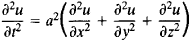Other examples are the corresponding inhomogeneous equations (in the right hand side of which known functions are added), such as the telegrapher’s equation. Equations and systems of this type appear in the analysis of various oscillations and wave processes. The properties of hyperbolic equations and systems are similar in many respects to the properties of the simple examples of such equations mentioned above.

The simplest elliptic equation is Laplace’s differential equation: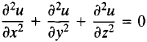Its inhomogeneous analogue is the Poisson equation. Elliptic equations and systems usually appear in the analysis of steady states.

A simple example of a parabolic equation is the heat equation: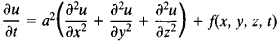Parabolic equations and systems usually appear in the analysis of equalizing processes.

The first example of an equation of mixed type was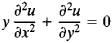Known as the Tricomi equation, it is elliptic in the half plane y > 0 (– ∞ < x < ∞), hyperbolic in the half plane y < 0, and parabolic along the straight line y = 0.

A number of problems of mathematical physics lead to various types of integral equations. For example, Volterra integral equations arise in those physics problems in which there is a preferential direction of change of an independent variable, such as time or energy. An integro-differential equation arises, in the problem of torsional oscillations.

Problem formulation and methods of solution of equations. In the first stage of development of the theory of equations of mathematical physics, much effort was expended on searching for a general solution. J. d’Alembert obtained in 1747 a general solution of the wave equation. On the basis of substitutions proposed by L. Euler in 1770, P. Laplace set forth in 1773 the “cascade method,” which gives a general solution of some other second-order linear homogeneous hyperbolic equations in two independent variables.

Such a general solution, however, has been found only in extremely rare cases. In contrast to ordinary differential equations, not a single important class of partial differential equations has been identified for which a general solution can be obtained in the form of a simple formula. Furthermore, it has turned out that in the analysis of physical processes the equations of mathematical physics usually appear together with additional conditions whose character fundamentally influences the direction in which a solution is sought (seeBOUNDARY VALUE PROBLEMS and CAUCHY PROBLEM).

Methods for the approximate solution of boundary value problems have come into wide use. In these methods the problem reduces to solving a system of algebraic (usually linear) equations (seeRITZ AND GALERKIN METHODS and NET-POINT METHOD). By increasing the number of unknowns in the system, the approximation can be carried out to any desired degree of accuracy.

### REFERENCES

Vladimirov, V. S. Uravneniia matematicheskoi fiziki, 2nd ed. Moscow, 1971.
Godunov, S. K. Uravneniia matematicheskoi fiziki. Moscow, 1971.
Sobolev, S. L. Uravneniia matematicheskoi fiziki, 4th ed. Moscow, 1966.
Tikhonov, A. N., and A. A. Samarskii. Uravneniia matematicheskoi fiziki, 4th ed. Moscow, 1972.
The Great Soviet Encyclopedia, 3rd Edition (1970-1979). © 2010 The Gale Group, Inc. All rights reserved.
References in periodicals archive ?
Shabat, "A scheme for integrating the nonlinear equations of mathematical physics by the method of the inverse scattering problem.
Blow-Up in Nonlinear Equations of Mathematical Physics: Theory and Methods
Fakhri, "Supersymmetry and shape invariance in differential equations of mathematical physics," Physics letters A, vol.
The existence of situations when one can map the dipole equation in traditional equations of mathematical physics (Bessel, confluent hypergeometric, Mathieu, etc.) by neglecting higher or lower powers in 1/a has a deep meaning.
Gliner, Differential Equations of Mathematical Physics, North-Holland, New York, NY, USA, 1964.
They cover elements of Hilbert space theory, Sobolev lattices, linear partial differential equations with constant coefficients, linear evolution equations, some evolution equations of mathematical physics, a royal road to initial boundary value problems.
The major themes addressed are hyperbolic geometry, automorphic functions, and applications to number theory; ordinary differential equations and dynamical systems; celestial mechanics and related problems; functions of several complex variables; algebraic topology and the Poincare conjecture; partial differential equations of mathematical physics; probability; continuous groups (Lie groups) and their algebras; the principle of relativity and the Poincare group; applied physics; and the philosophy of science.
Serova, The conditional symmetry and connection between the equations of mathematical physics, Symmetry in Nonlinear Mathematical Physics 1 (1997), pp.
FAKHRI, Parasupersymmetry and shape invariance in differential equations of mathematical physics and quantum mechanics, Ann.
Lewy, On the partial difference equations of mathematical physics, IBM J.

Site: Follow: Share:
Open / Close